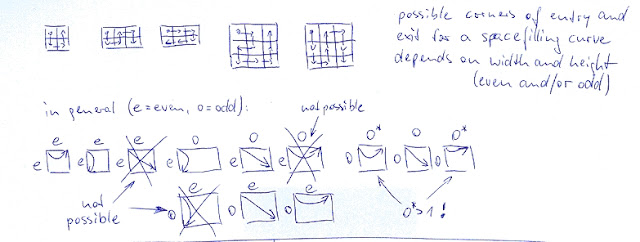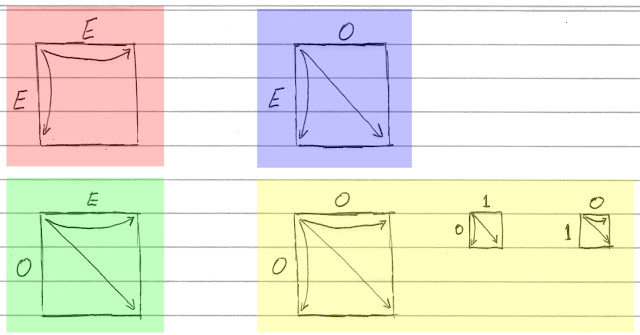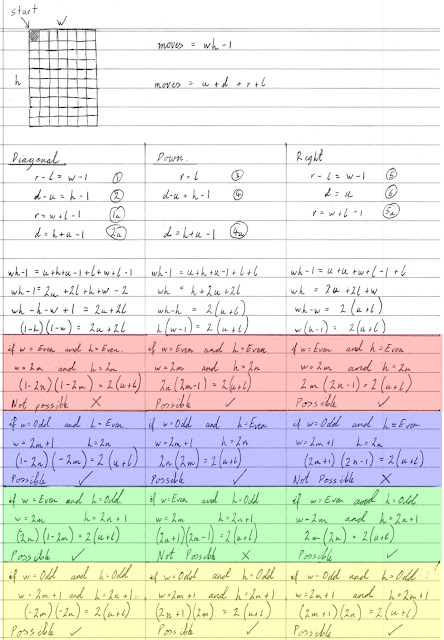## Saturday, February 11, 2017

### I hope you find this blog enjoyable and helpful. Support it with a small donation.

I've been researching space filling curves recently and came across an interesting algorithm to generate curves for arbitrary areas. To be completely accurate, space filling curves cover the entire unit square in continuous space, whereas what I'm looking at is the discrete form that covers a grid. Below when a path or curve is mentioned, what is meant is a way to cover a rectangular area by moving one square at a time, either up, down, left, or right, without crossing over itself. The curve starts in one corner and ends in another.

Being almost completely new to this, I set about understanding the description of the algorithm. Although it was accompanied by code, details of the process were scant. The first part described rules for valid corner exit points given a starting point on grids with edges that are either odd or even. This is what I want to explore in this blog post.

A rectangular area can have an either odd or even width and an either odd or even height. There are 4 possible combinations of these edge dimensions and for each one, a set of directions were supplied. I didn't just want to take these at face value. Some sort of proof was needed.Space Filling Curve Algorithm by Lutz Tautenhahn

The proof below doesn't say anything about the existence of a path/curve that starts at one corner and ends at another covering all squares. All it does is show if a path is possible or not. Although it's relatively easy to prove that a path can be found for all of the scenarios below, we'll leave that for another time.

So what are the results?  In grids with two even edges, a path can be found from a corner to either adjacent corner, but not to the diagonal corner.  In grids with an even and odd side a path can be found to the diagonal corner and the adjacent corner on the even side but not the odd side.  Finally in a grid with two odd sides a path can be found to any other corner except if one of the odd edges is equal to 1.  In that case the path is only diagonal or along the odd side that isn't one.  That last bit isn't in the proof but it's obvious.Allow directions in a space filling grid

Proving the directions is straight forward. If you imagine that the corner start square of a grid is covered, and every time you move, another square is covered, it becomes obvious that there are (wh-1) moves until the entire space is covered. The total number of moves is also equal to the number of up, down, left, and right moves. If you want to move right side of the grid the number of right moves minus the number of left moves has to be equal to (w-1). If you want to stay on the left, the number of left moves is equal to the number of right moves.  Similarly if you want to move to the bottom of the grid, the number of down moves minus the number of up moves is equal to (h-1). If you want to stay at the top, the number of down and up moves mus t be equal. When these equations are combined the directions above can be found.Grid movement proof

It was redundant to prove both the Odd-Even and Even-Odd case as they are a diagonal mirroring of the same scenario.  It's also sufficient to only show the paths starting at the top left corner as paths starting in other corners are vertical and or horizontal mirrors of paths in the top left corner.

Although I didn't end up using the curve filling path in the first image, it helped me understand some of the subtleties of curve generation.